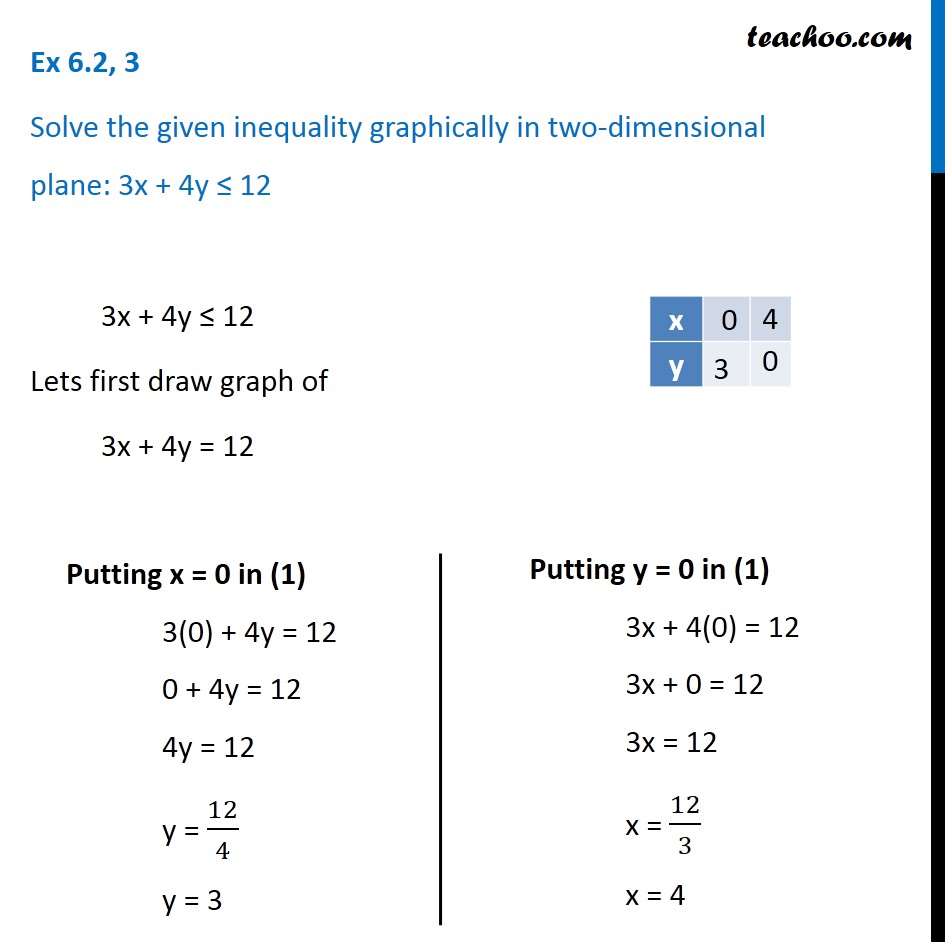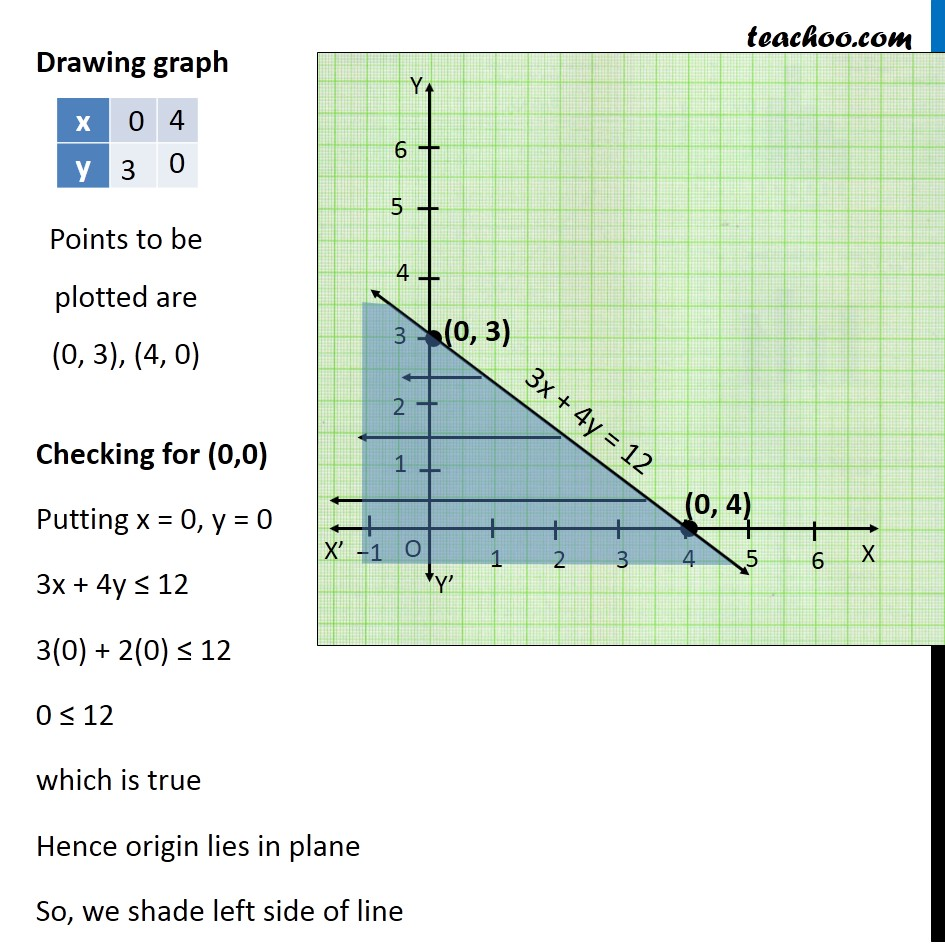Solving Linear Inequality Graphically

Chapter 5 Class 11 Linear Inequalities
Serial order wiseLearn in your speed, with individual attention - Teachoo Maths 1-on-1 Class

### Transcript

Question 3 Solve the given inequality graphically in two-dimensional plane: 3x + 4y ≤ 12 3x + 4y ≤ 12 Lets first draw graph of 3x + 4y = 12 Putting x = 0 in (1) 3(0) + 4y = 12 0 + 4y = 12 4y = 12 y = 12/4 y = 3 Putting y = 0 in (1) 3x + 4(0) = 12 3x + 0 = 12 3x = 12 x = 12/3 x = 4 Drawing graph Checking for (0,0) Putting x = 0, y = 0 3x + 4y ≤ 12 3(0) + 2(0) ≤ 12 0 ≤ 12 which is true Hence origin lies in plane So, we shade left side of line Points to be plotted are (0, 3), (4, 0)